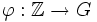Tour:Equivalence of definitions of cyclic group

PREVIOUS: Cyclic group| UP: Introduction four (beginners)| NEXT: Every nontrivial subgroup of the group of integers is cyclic on its smallest element
General instructions for the tour | Pedagogical notes for the tour | Pedagogical notes for this part
WHAT YOU NEED TO DO:
• Read, and thoroughly understand, the equivalence of the two definitions of cyclic group. (We'll see better formulations of the proof later, after introducing homomorphisms and quotients)

The definitions that we have to prove as equivalent

Definition in terms of modular arithmetic

A group is said to be cyclic (sometimes, monogenic or monogenous) if it is either isomorphic to the group of integers or to the group of integers modulo n for some positive integer$n$.

Definition in terms of generating sets

A group is termed cyclic (sometimes, monogenic or monogenous) if it has a generating set of size 1.

Proof of equivalence

From modular arithmetic to generating sets

This is direct:$\mathbb{Z}$ is generated by the element$1 \in \mathbb{Z}$, and$\mathbb{Z}/n\mathbb{Z}$ is generated by the element 1.

From generating sets to modular arithmetic

Given: A group$G$ with a generating set$\{ g \}$

To prove:$G$ is isomorphic either to$\mathbb{Z}$ (the group of integers) or to$\mathbb{Z}/n\mathbb{Z}$ (the group of integers modulo n)

Proof: We consider two cases.

Case 1:$g$ has finite order. Thus, there exists a minimal positive integer$n$ such that$g^n$ is the identity element. Consider now the map$\varphi: \mathbb{Z}/n\mathbb{Z} \to G$ that sends$a$ to the element$g^a$. We want to prove that$\varphi$ is an isomorphism.

We first show that$\varphi(a + b) = \varphi(a)\varphi(b)$. For this, observe that if$a$ and$b$ add up to less than$n$ as integers, then$g^{a+b} = g^ag^b$ by definition. If the sum of$a$ and$b$ as integers is at least$n$, then$\varphi(a + b) = g^{a+b-n} =g^ag^bg^{-n} = g^ag^b$ (since$g^{-n}$ is the identity element).

Similarly,$\varphi(0) = g^0 = e$ by definition, and$\varphi(-a) = \varphi(a)^{-1}$, again because$g^n = e$.

Surjectivity: Since$g$ generates$G$, every element of$G$ can be written as a power of$g$, say$g^m$ for some integer$m$. Writing$m = nq + r$ where$q,r$ are integers and$r \in \{ 0,1,2,\dots,n-1 \}$, we get that$g^m = g^r = \varphi(r)$. Thus,$\varphi$ is surjective.

Injectivity: Finally, if$\varphi(a) = \varphi(b)$ with$a < b$ both in$\{ 0,1,2,\dots,n-1 \}$, then$g^{b - a} = e$, contradicting the assumption that$g$ has order$n$.

Thus,$\varphi$ is an isomorphism of groups.

Case 2:$g$ does not have finite order. In that case, consider the map$\varphi:\mathbb{Z} \to G$ that sends$n$ to$g^n$.

Clearly, by definition,$\varphi(a + b) = \varphi(a)\varphi(b)$,$\varphi(-a) = \varphi(a)^{-1}$, and$\varphi(0) = e$.

Surjectivity: Since$g$ generates$G$, every element of$G$ can be written as$g^n$ for some integer$n$.

Injectivity: If$\varphi(a) = \varphi(b)$ for$a < b$, then$g^{b - a} = e$, contradicting the assumption that$g$ does not have finite order.